Courses

# Notes | EduRev

## NEET : Notes | EduRev

The document Notes | EduRev is a part of the NEET Course Physics 28 Years Past year papers for NEET/AIPMT Class 12.
All you need of NEET at this link: NEET

Q.1. Pick the wrong answer in the context with rainbow.    (2019)
A: When the light rays undergo two internal reflections in a water drop, a secondary rainbow is formed
B: The order of colours is reversed in the secondary rainbow
C: An observer can see a rainbow when his front is towards the sun
D: Rainbow is a combined effect of dispersion refraction and  reflection of sunlight
Ans:
C
Solution:

Rainbow can't be observed when observer faces towards sun.

Q.2. Two similar thin equi-convex lenses, of focal length f each, are kept coaxially in contact with each other such that the focal length of the combination is F1. When the space between the two lenses is filled with glycerine (which has the same refractive index (μ = 1.5) as that of glass) then the equivalent focal length is F2. The ratio F1 :  F2 will be :    (2019)
A: 2 : 1
B: 1 : 2
C: 2 : 3
D: 3 : 4
Ans:
B
Solution: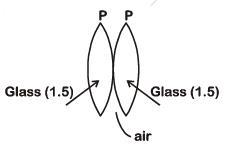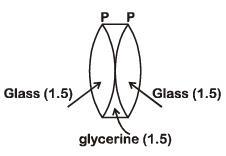Equivalent focal length in air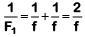When glycerin is filled inside, glycerin lens behaves like a diverging lens of focal length (-f)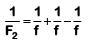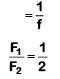Q.3. In total internal reflection when the angle of incidence is equal to the critical angle for the pair of media in contact, what will be angle of refraction?    (2019)
A: 180°
B: 0°
C: Equal to angle of incidence
D: 90°
Ans:
D
Solution:

At i = ic, refracted ray  grazes  with the surface.
So angle of refraction is 90°.

Q.4. The refractive index of the material of a prism is √2 and the angle of the prism is 30°. One of the two refracting surfaces of the prism is made a mirror inwards, by silver coating. A beam of monochromatic light entering the prism from the other face will retrace its path (after reflection from the silvered surface) if its angle of incidence on the prism is :-    (2018)
A: 60°
B: 45°
C: 30°
D: zero
Ans:
B
Solution: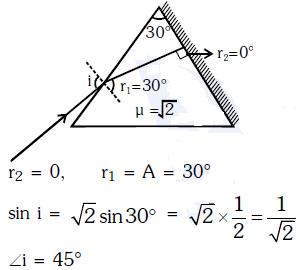Q.5. An object is placed at a distance of 40 cm from a concave mirror of focal length 15 cm. If the object is displaced through a distance of 20 cm to wards the mirror, the displacement of the image will be:-    (2018)
A: 30 cm away from the mirror
B: 36 cm away from the mirror
C: 30 cm towards the mirror
D: 36 cm towards the mirror
Ans:
B
Solution: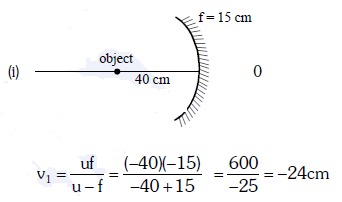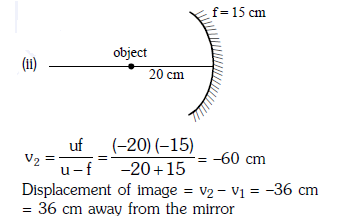Q.6. An astronomical refracting telescope will have large angular magnification and high angular resolution, when it has an objective lens of :-    (2018)
A: small focal length and large diameter
B: large focal length and small diameter
C: large focal length and large diameter
D: small focal length and small diameter
Ans: C
Solution:

For astronomical refracting telescope
Angular magnification is for large focal length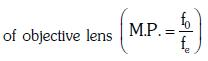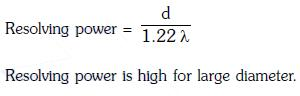Q.7. A beam of light from a source L is incident normally on a plane mirror fixed at a certain distance x from the source. The beam is reflected back as a spot on a scale placed just above the source I. When the mirror is rotated through a small angle q, the spot of the light is found to move through a distance yon the scale. The angle q is given by :-   (2017)
A: y/x
B: x/2y
C: x/y
D: y/2x

Ans: D
Solution: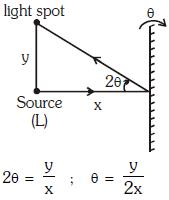Q.8. The ratio of resolving powers of an optical microscope for two wave lengths λ1 = 4000 Å and λ2 = 6000 Å is :-    (2017)
A: 9 : 4
B: 3 : 2
C: 16 : 81
D: 8 : 27
Ans:
B
Solution: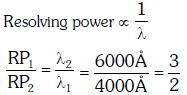Q.9. A thin prism having refracting angle 10° is made of glass of refractive index 1.42. This prism is combined with another thin prism of glass of refractive index 1.7. This combination produces dispersion without deviation. The refracting angle of second prism should be :-    (2017)
A: 6°
B: 8°
C: 10°
D: 4°
Ans:
A
Solution:

(μ -1)A + (μ'-1)A = 0
(μ-1)A |(μ'-1)A'|
(1.42 -1)x10° = (1.7 -1)A'
4.2 = 0.7A'
A' = 6°

Q.10. A astronomical telescope has objective and eyepiece of focal lengths 40 cm and 4 cm respectively. To view an object 200 cm away from the objective, the lenses must be separated by a distance :    (2016)
A: 54 cm
B: 37.3 cm
C: 46 cm
D: 50 cm
Ans: A
Solution:

According to a question,
Focal length of the objective lens, Fo = +40 cm
Focal length of eyepiece, Fe = 4 cm
Object distance for objective lens (uo) = -200 cm
Applying lens formula for objective lens,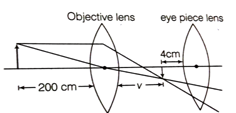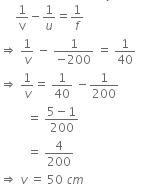Image will be formed at the focus of a eyepiece lens.
So, for normal adjustment distance between objectives and eyepiece (length of tube) will be, v + Fe = 50 + 4 = 54 cm

Q.11. Match the corresponding entries of column-1 with column-2. [Where m is the magnification produced by the mirror]     (2016)
Column - 1                   Column - 2

(A) m = -2              (a) Convex mirror
(B) m = -1/2          (b) Concave mirror
(C) m = +2             (c) Real image
(D) m = =1/2         (d) Virtual image
A: A → c and d; B → b and d; C → b and c; D → a and d
B: A → b and c; B → b and c; C → b and d; D → a and d
C: A → a and c; B → a and d; C → a and b; D → c and d
D: A → a and d; B → b and c; C → b and d; D → b and c
Ans:
B
Solution:

(A) m = -2, so image is magnified and inverted. Which is possible only for concave mirror. since image is i inverted so it will be real.
(B) M = -1/2 , so image is inverted and diminished. since image is inverted, so it will be real, and the mirror will be concave.
(C) M = +2, image is magnified so the mirror will be concave. Image is erect so it will be virtual.
(D) m = +1/2 , image is erect so image will be virtual. Image is virtual and diminished, so the mirror should be convex.

Q.12. The angle incidence for a ray of light at a refracting surface of a prism is 45 degree.The angle of prism is 60 degree If the ray suffers minimum deviation through the prism, the angle of minimum deviation and refractive index of the material of the prism respectively, are     (2016)
A: 30 deg; 1/√2
B: 45 deg; 1/√2
C: 30 deg; √2
D: 45 deg; √2
Ans:
C
Solution: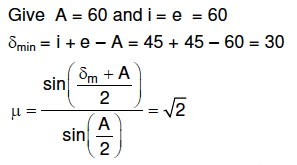Q.13.  The refracting angle of a prism is A, and refractive index of the material of the prism is cot (A/2). The angle of minimum deviation is :     (2015)
A: 180º + 2A
B: 180º - 3A
C: 180º - 2A
D: 90º - A
Ans:
C
Solution: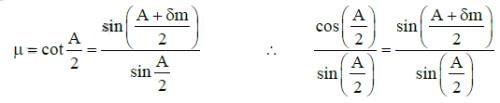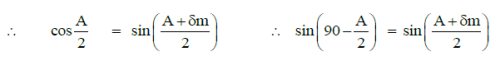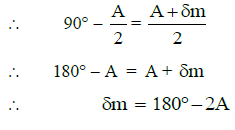Q.14. Two identical thin plano-convex glass lenses (refractive index 1.5) each having radius of curvature of 20 cm are placed with their convex surfaces in contact at the centre. The intervening space is filled with oil of refractive index 1.7. The focal length of the combination is :     (2015)
A: 50 cm
B: -20 cm
C: -25 cm
D: -50 cm
Ans:
D
Solution: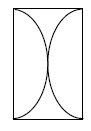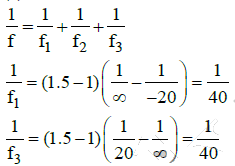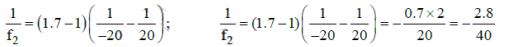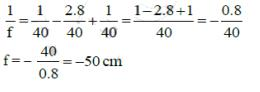Q.15. The angle of a prism is ‘A’. One of its refracting surfaces is silvered. Light rays falling at an angle of incidence 2A on the first surface returns back through the same path after suffering reflection at the silvered surface. The refractive index μ, of the prism is :     (2014)
A: 1/2 cos A
B: tan A
C: 2 sin A

D: 2 cos A
Ans:
D
Solution: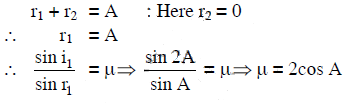Q.16. If the focal length of objective lens is increased then magnifying power of :      (2014)
A: microscope and telescope both will decrease
B: microscope will decrease but that of telescope will increase
C: microscope will increase but that of telescope will decrease
D: microscope and telescope both will increase
Ans:
B
Solution: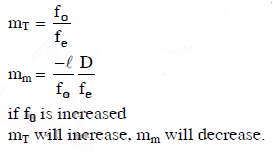Offer running on EduRev: Apply code STAYHOME200 to get INR 200 off on our premium plan EduRev Infinity!

,

,

,

,

,

,

,

,

,

,

,

,

,

,

,

,

,

,

,

,

,

;